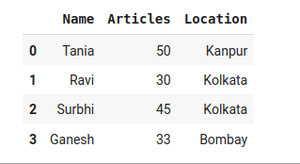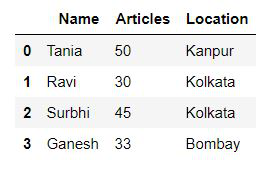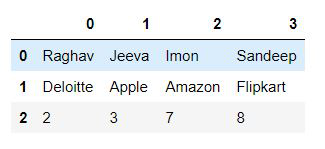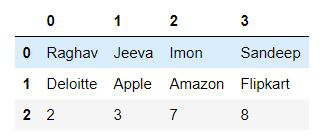# Align columns to Left in Pandas – Python

Pandas library is useful for performing exploratory data analysis in Python. A pandas dataframe represents data in a tabular format. We can perform operations on the data and display it. In this article, we are going to align columns to the Left in Pandas. When we display the dataframe, we can align the data in the columns as left, right, or center.

The default is right alignment as we can see in the below example.

## Python3

 `# Python code demonstrate creating ` `# DataFrame from dict and left aligning` `import` `pandas as pd `   `# initialise data of lists. ` `data ``=` `{``'Name'` `: [``'Tania'``, ``'Ravi'``, ` `                  ``'Surbhi'``, ``'Ganesh'``], ` `        `  `        ``'Articles'` `: [``50``, ``30``, ``45``, ``33``], ` `        `  `        ``'Location'` `: [``'Kanpur'``, ``'Kolkata'``,` `                      ``'Kolkata'``, ``'Bombay'``]} `   `# Create DataFrame ` `df ``=` `pd.DataFrame(data) ` `display(df)`

Output:In order to align columns to left in pandas dataframe, we use the dataframe.style.set_properties() function.

Syntax: Styler.set_properties(subset=None, **kwargs)

Parameters:

• subsetIndexSlice: A valid slice for data to limit the style application to.
• **kwargsdict: A dictionary of property, value pairs to be set for each cell.

Returns: selfStyler

Example 1:

## Python3

 `# Python code demonstrate creating ` `# DataFrame from dict and left aligning` `import` `pandas as pd `   `# initialise data of lists. ` `data ``=` `{``'Name'` `: [``'Tania'``, ``'Ravi'``, ` `                  ``'Surbhi'``, ``'Ganesh'``], ` `        `  `        ``'Articles'` `: [``50``, ``30``, ``45``, ``33``], ` `        `  `        ``'Location'` `: [``'Kanpur'``, ``'Kolkata'``,` `                      ``'Kolkata'``, ``'Bombay'``]} `   `# Create DataFrame ` `df ``=` `pd.DataFrame(data) `   `left_aligned_df ``=` `df.style.set_properties(``*``*``{``'text-align'``: ``'left'``})` `display(left_aligned_df)`

Output:Example 2:

## Python3

 `import` `pandas as pd `   `# initialise data of lists. ` `data ``=` `[[``'Raghav'``, ``'Jeeva'``, ``'Imon'``, ``'Sandeep'``], ` `        ``[``'Deloitte'``, ``'Apple'``, ``'Amazon'``, ``'Flipkart'``], ` `        ``[``2``,``3``,``7``,``8``]]`   `# Create DataFrame ` `df ``=` `pd.DataFrame(data) ` `left_aligned_df ``=` `df.style.set_properties(``*``*``{``'text-align'``: ``'left'``})` `display(left_aligned_df)`

Output:In the above example, the content of all columns are left-aligned, except the column headers. The column headers are center-aligned.

Example 3:

If we want the column headers aligned left, we use the set_table_styles() function.

## Python3

 `import` `pandas as pd `   `# initialise data of lists. ` `data ``=` `[[``'Raghav'``, ``'Jeeva'``, ``'Imon'``, ``'Sandeep'``],` `        ``[``'Deloitte'``, ``'Apple'``, ``'Amazon'``, ``'Flipkart'``],` `        ``[``2``,``3``,``7``,``8``]]`   `# Create DataFrame ` `df ``=` `pd.DataFrame(data) ` `left_aligned_df ``=` `df.style.set_properties(``*``*``{``'text-align'``: ``'left'``})`   `left_aligned_df ``=` `left_aligned_df.set_table_styles(` `[``dict``(selector ``=` `'th'``, props``=``[(``'text-align'``, ``'left'``)])])`   `display(left_aligned_df)`

Output:In the above example, both the column headers and the content of all the columns are left-aligned.

Whether you're preparing for your first job interview or aiming to upskill in this ever-evolving tech landscape, GeeksforGeeks Courses are your key to success. We provide top-quality content at affordable prices, all geared towards accelerating your growth in a time-bound manner. Join the millions we've already empowered, and we're here to do the same for you. Don't miss out - check it out now!

Previous
Next